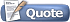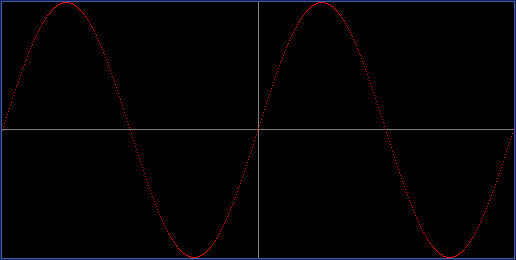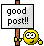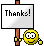Welcome to Doom9's Forum, THE in-place to be for everyone interested in DVD conversion. Before you start posting please read the forum rules. By posting to this forum you agree to abide by the rules.Doom9's ForumBraindead Folly
 User Name Remember Me? Password
 Register FAQ Calendar Search Today's Posts Mark Forums Read

 Thread Tools Search this Thread Display Modes17th October 2021, 16:39 #1  |  Link StainlessS HeartlessS Usurer   Join Date: Dec 2009 Location: Over the rainbow Posts: 10,260 Braindead Folly Hi guys, can you math geniuses out there try this thingy out and point out any screwups I've made [if any]. A daft script I've spent a couple of days on, a bit experimental and will tend to crash due to stack exhaustion when over doing it plotting points on graph. Anyways, here tis. Code: ```/* Silly folly. GrafCanvas(), Make a single frame graph clip. GrafFunc(), Plot an avisynth function, via Eval. GrafFunc_mt_Lut() Plot mt_lut expression. GrafCanvas, create single frame graph clip. [Prone to crash due to stack exhaustion if too many pixels plotted] xmax, ymax; specify maximum physical pixel coords, range of graf 0->xmax, 0->ymax. Logical coords are used only by GrafFunc() function, eg GrafFunc("sin(x-PI)",color=\$FF0000). Logical coords specified by startx/y and rangex/y. If start and range not specified, then logical and physical coords are the same, 0->xmax, 0->ymax. Examples: Plot sin() in red, 2 cycles centered both x and y, with origin axis shown at 0,0. GrafCanvas(511,255,startx=-2*PI, rangex=4*PI, starty=-1.0, rangey=2.0).GrafFunc("sin(x)",\$FF0000) About the same but with origin axis shifted off center. GrafCanvas(511,255,startx =-PI, rangex=4*PI, starty=-1.0, rangey=2.0).GrafFunc("sin(x)",\$FF0000) 3 Phase [Crash on my machine if 511,255, too many points plotted, stack overflow or something] GrafCanvas(255,255,startx=-2*PI,rangex=4*PI,starty=-1.0,rangey=2.0).GrafFunc("sin(x)",\$FF0000).GrafFunc("sin(x-2*PI/3)",\$00FF00).GrafFunc("sin(x-4*PI/3)",\$0000FF) 4 Phase [AXIS OFF else Crash] GrafCanvas(255,255,startx=-2*PI,rangex=4*PI,starty=-1.0,rangey=2.0,AXIS=FALSE).GrafFunc("sin(x)",\$FF0000).GrafFunc("sin(x-1*PI/2)",\$00FF00).GrafFunc("sin(x-2*PI/2)",\$0000FF).GrafFunc("sin(x-3*PI/2)",\$FF00FF) Suggest load into Vdub2, right click and tick "Free Adjust", then double click the graphic. */ # Examples GrafCanvas(511,255,startx=-2*PI, rangex=4*PI, starty=-1.0, rangey=2.0).GrafFunc("sin(x)",\$FF0000) #GrafCanvas(511,255,startx =-PI, rangex=4*PI, starty=-1.0, rangey=2.0).GrafFunc("sin(x)",\$FF00FF) #GrafCanvas(255,255,startx=-2*PI,rangex=4*PI,starty=-1.0,rangey=2.0).GrafFunc("sin(x)",\$FF0000).GrafFunc("sin(x-2*PI/3)",\$00FF00).GrafFunc("sin(x-4*PI/3)",\$0000FF) #GrafCanvas(255,255,startx=-2*PI,rangex=4*PI,starty=-1.0,rangey=2.0,AXIS=FALSE).GrafFunc("sin(x)",\$FF0000).GrafFunc("sin(x-1*PI/2)",\$00FF00).GrafFunc("sin(x-2*PI/2)",\$0000FF).GrafFunc("sin(x-3*PI/2)",\$FF00FF) #GrafFunc_mt_Lut("x") # Infix "x" #GrafFunc_mt_Lut("255 x -") # Infix "255 - x" #GrafFunc_mt_Lut("x 16 < x 235 > | 0 255 ?") # Infix "(x < 16 | x > 235) ? 0 : 255" #GrafFunc_mt_Lutxy() # Infix = "x" (default) #GrafFunc_mt_Lutxy("x y +",128) # Infix = "x + y" #GrafFunc_mt_Lutxy("x y -",128) # Infix = "x - y" #GrafFunc_mt_Lutxy("255 x y - abs 16 * -", y_Y = 0) # Infix = "255-(abs(x-y)*16)" #GrafFunc_mt_Lutxy("255 x y - abs 16 * -", y_Y=128) # Infix = "255-(abs(x-y)*16)" #GrafFunc_mt_Lutxy("255 x y - abs 16 * -", y_Y=255) # Infix = "255-(abs(x-y)*16)" #GrafFunc_mt_Lutxy("x y - abs 128 / 1 4.0 x y - abs 64 / - / ^ 255 *", y_Y = 0) # Infix="( (abs(x-y)/128) ^ (1/ (4.0-(abs(x-y)/64)) ) )*255" #GrafFunc_mt_Lutxy("x y - abs 128 / 1 4.0 x y - abs 64 / - / ^ 255 *", y_Y=128) # Infix="( (abs(x-y)/128) ^ (1/ (4.0-(abs(x-y)/64)) ) )*255" #GrafFunc_mt_Lutxy("x y - abs 128 / 1 4.0 x y - abs 64 / - / ^ 255 *", y_Y=255) # Infix="( (abs(x-y)/128) ^ (1/ (4.0-(abs(x-y)/64)) ) )*255" #GrafFunc_mt_Lutxyz("x y - abs 128 / 1 4.0 x y - abs 64 / - / ^ 255 *", y_Y=128, z_Y= 128) # Infix="( (abs(x-y)/128) ^ (1/ (4.0-(abs(x-y)/64)) ) )*255" [z NOT used, same as above GrafFunc_mt_Lutxy] #GrafFunc_mt_Lutxyz("y x - 2.0 ^ z x - 2.0 ^ + 0.5 ^", y_Y=128, z_Y= 128) # Infix="(((y-x)^2.0)+((z-x)^2.0))^0.5" #GrafFunc_mt_Lutxyz("y x - 2.0 ^ z x - 2.0 ^ + 0.5 ^", y_Y=64, z_Y= 64) # Infix="(((y-x)^2.0)+((z-x)^2.0))^0.5" #GrafFunc_mt_Lutxyz("y x - 2.0 ^ z x - 2.0 ^ + 0.5 ^", y_Y=192, z_Y= 192) # Infix="(((y-x)^2.0)+((z-x)^2.0))^0.5" #GrafFunc_mt_Lutxyz("y x - 2.0 ^ z x - 2.0 ^ + 0.5 ^", y_Y=64, z_Y= 192) # Infix="(((y-x)^2.0)+((z-x)^2.0))^0.5" #GrafFunc_mt_Lutxyz("255 y x - 2.0 ^ z x - 2.0 ^ + 0.5 ^ -", y_Y=64, z_Y= 192) # Infix="255-((((y-x)^2.0)+((z-x)^2.0))^0.5)" #GrafFunc_mt_Lutxyz("y x - 2.0 ^ z x - 2.0 ^ + 0.5 ^", y_Y=32, z_Y= 224) # Infix="(((y-x)^2.0)+((z-x)^2.0))^0.5" #GrafFunc_mt_Lutxyz("255 y x - 2.0 ^ z x - 2.0 ^ + 0.5 ^ -", y_Y=32, z_Y= 224) # Infix="255-((((y-x)^2.0)+((z-x)^2.0))^0.5)" return last Function GrafCanvas(int "xmax",int "ymax", [* Physical pixel max coords (suggest 255) *] \ float "startx",float "rangex",float "starty",float "rangey", [* Logical coords bottom left, and ranges, eg startx=-PI, rangex=2*PI, starty=-1.0, Rangey=2.0 *] \ int "bkColor",int "iColor",int "oColor",int "aColor",int "pColor", \ bool "Axis" [* May need to switch off Axis if crash *] \ ) { xmax = Default(xmax,255) ymax = Default(ymax,255) # Max Physical X and Y pixel coords of drawing area. [physical xmin,ymin are always 0] startx = Default(startx,0) rangex = Default(rangex,xmax) # Leftmost logical x coord and range to logical RHS pixel. starty = Default(starty,0) rangey = Default(rangey,ymax) # Bottommost logical y coord and range to logical Topmost pixel. bkColor= default(bkColor,\$000000).BitAnd(\$FFFFFF) # Background color of drawing area. iColor = default(iColor, \$4060A0).BitAnd(\$FFFFFF) # Inner perimeter color outside of drawing area. oColor = default(oColor ,\$102060).BitAnd(\$FFFFFF) # Outer perimeter color, outside of Inner perimeter. aColor = default(aColor ,\$808080).BitAnd(\$FFFFFF) # Axis color. pColor = default(pColor ,\$FFFFFF).BitAnd(\$FFFFFF) # Plotted pixel color. axis = Default(axis,true) # Draw Axis. Global G_xmax = xmax Global G_ymax = ymax # *** GLOBALS *** Global G_startx = startx Global G_rangex = rangex Global G_starty = starty Global G_rangey = rangey Global G_pColor = pColor physicalx = (startx==0 && rangex==xmax) physicaly = (starty==0 && rangey==ymax) BlankClip(Pixel_type="RGB32",Length=1,Width=xmax+1,Height=ymax+1,Color=bkColor) Addborders(1,1,1,1,iColor) Addborders(1,1,1,1,oColor) if(axis) { if(physicalx) { axis_x=(xmax/2.0).Round } else { if(startx <= 0 <= (startx + rangex)) { axis_x = (xmax * (0.0 - startx) / rangex).Round } else { axis_x = - 1 } } if(0 <= axis_x <= xmax) { for(y=0,ymax) { GrafPoint(axis_x,y,color=aColor) } } if(physicaly) { axis_y=(ymax/2.0).Round } else { if(starty <= 0 <= (starty + rangey)) { axis_y = (ymax * (0.0 - starty) / rangey).Round } else { axis_y = - 1 } } if(0 <= axis_y <= ymax) { for(x=0,xmax) { GrafPoint(x,axis_y,color=aColor) } } } Return Last } Function GrafPoint(clip c,int x,int y,int "Color") { # Physical coords plotter. c W = Width H=Height Color = default(Color,G_pColor) xmax = W-5 ymax = H-5 if(!(0 <= x <= xmax && 0 <= y <= ymax)) { RT_DebugF("Coord out of Bounds, x=%d y=%d",x,y,name="GrafPoint: ") x=x.min(xmax).max(0) y=y.min(ymax).max(0) } pix = Last.BlankClip(Pixel_Type="RGB32",Length=1,width=1,height=1,color=Color) xo = 2 xr = xo + x yo = H-3 yr = yo - y Overlay(pix,x=xr,y=yr,opacity=1.0) Return Last } Function GrafFunc(clip c,String func,int "Color") { # eg GrafFunc(grafclip,"sin(x-PI/4)",color=\$FFFFFF) ie sine function delayed by 45 degrees (PI/4 radians). c xstepf = G_rangex/G_xmax ystepf = G_rangey/G_ymax startx = G_startx starty = G_starty for(xi=0, G_xmax) { x = xi * xstepf + startx y = Eval(func) yi = (((y - starty) / ystepf)).Round GrafPoint(xi,yi,Color) } } Function GrafFunc_mt_Lut(String "yexpr",int "Color") { RT_DebugF("DBGVIEWCLEAR",name="CLEAR DEBUGVIEW: ") yexpr = Default(yexpr,"x") Color = Default(Color,\$FF00FF) GrafCanvas(255,255) x_C = BlankClip(width=256,height=1,length=1,Pixel_type="Y8").mt_lutspa(mode = "absolute", expr = "x") R_C = X_C.Mt_lut(yexpr=yexpr) RT_DebugF("YEXPR='%s' :: INFIX='%s'",yexpr,mt_Infix(yexpr),name="GrafFunc_mt_Lut: ") for(x=0,255) { Y=R_C.RT_AverageLuma(n=0,x=x,y=0,w=1,h=1).Round GrafPoint(x,y,color=Color) } Return Last } Function GrafFunc_mt_Lutxy(String "yexpr",Int "y_Y",int "Color") { # y_Y 0->255 RT_DebugF("DBGVIEWCLEAR",name="CLEAR DEBUGVIEW: ") yexpr = Default(yexpr,"x") y_Y = Default(y_Y, 128) Color = Default(Color,\$FF00FF) GrafCanvas(255,255) Y_C = BlankClip(Pixel_type="Y8",Length=1,Width=4,Height=4,Color_YUV=(y_Y *256+128)*256+128) X_C = BlankClip(width=256,height=1,length=1,Pixel_type="Y8").mt_lutspa(mode = "absolute", expr = "x") R_C=Mt_lutxy(X_C,Y_C,yexpr=yexpr) RT_DebugF("YEXPR='%s' :: INFIX='%s'",yexpr,mt_Infix(yexpr),name="GrafFunc_mt_Lutxy: ") for(x=0,255) { Y=R_C.RT_AverageLuma(n=0,x=x,y=0,w=1,h=1).Round GrafPoint(x,y,color=Color) } Return Last } Function GrafFunc_mt_Lutxyz(String "yexpr",Int "y_Y",Int "z_Y",int "Color") { # y_Y and z_Y, 0->255 RT_DebugF("DBGVIEWCLEAR",name="CLEAR DEBUGVIEW: ") yexpr = Default(yexpr,"x") y_Y = Default(y_Y,128) z_Y = Default(z_Y,128) Color = Default(Color,\$FF00FF) GrafCanvas(255,255) Y_C = BlankClip(Pixel_type="Y8",Length=1,Width=4,Height=4, Color_YUV=(y_Y *256+128)*256+128) Z_C = BlankClip(Pixel_type="Y8",Length=1,Width=4,Height=4,Color_YUV=(z_Y *256+128)*256+128) X_C = BlankClip(width=256,height=1,length=1,Pixel_type="Y8").mt_lutspa(mode = "absolute", expr = "x") R_C = Mt_lutxyz(X_C,Y_C,Z_C,yexpr=yexpr) RT_DebugF("YEXPR='%s' :: INFIX='%s'",yexpr,mt_Infix(yexpr),name="GrafFunc_mt_Lutxyz: ") for(x=0,255) { Y=R_C.RT_AverageLuma(n=0,x=x,y=0,w=1,h=1).Round GrafPoint(x,y,color=Color) } Return Last }``` Try out the examples. [uncomment] Here, masktools lut tutorial by tp7:- https://tp7.github.io/articles/masktools/ You can try out some of the examples there. __________________ I sometimes post sober. StainlessS@MediaFire ::: AND/OR ::: StainlessS@SendSpace "Some infinities are bigger than other infinities", but how many of them are infinitely bigger ??? Last edited by StainlessS; 17th October 2021 at 20:53.17th October 2021, 17:00 #2  |  Link StainlessS HeartlessS Usurer   Join Date: Dec 2009 Location: Over the rainbow Posts: 10,260 Some of the examples,resized wider as crash due to stack exhaustion if done wider.One of the lut examples, also resized wider.__________________ I sometimes post sober. StainlessS@MediaFire ::: AND/OR ::: StainlessS@SendSpace "Some infinities are bigger than other infinities", but how many of them are infinitely bigger ??? Last edited by StainlessS; 17th October 2021 at 17:02.17th October 2021, 22:37 #3  |  Link johnmeyer Registered User   Join Date: Feb 2002 Location: California Posts: 2,583 What math? You are just plotting sin functions. Is there something mathematically complex that I am supposed to comment on? Or are you just looking for a way to make it less resource intensive (i.e., avoid crashes)?17th October 2021, 22:49 #4  |  Link Dogway Registered User   Join Date: Nov 2009 Posts: 2,004 Looks very cool, I also made a function graph a few weeks ago (work in progress though). I simply use a gradient and histogram to draw the lines. Not sure if it helps. I didn't finish the zoom part, also wanted to add x and y notations to the axes. Code: ```### ### Curve Fitting Evaluator ### ### Dependencies: ### ExTools ### manyplus ### ResizersPack ### ### Example: ### GradientLinear() ### # ex_contrast(cont=1) # Here my curve expression ### HistoCurve() ### ###################### function GradientLinear(clip a, float "zoom", bool "tv_range") { tv = Default(tv_range, false) zm = Default(zoom, 1) of = zm zm = zm * 2. a.ConverttoYUV420() BilinearResize(256,256) ConvertBits(32) !tv ? ex_lutspa(mode="relative", expr="x range_max *", UV=128) : \ ex_lutspa(mode="relative", expr="x range_max * ymax ymin - range_max / * ymin +", UV=128) Expr(Format("x {zm} * {of} -"),"") } function HistoCurve(clip a, float "zoom") { zm = Default(zoom, 1) grid=BlankClip(a).Grid(max(4,(round(26/zm)/2)*2),1,2,\$ffffff,\$ffffff,\$ffffff,grid=true,axis=true) of = zm zm = zm * 2. Expr(a,Format("x {of} + {zm} / "),"") TurnRight() Histogram(mode="classic") TurnLeft() hist=crop(0,0,0,-256).ex_lut("x range_half < 0 x ?", UV=128).ex_blend(grid,mode="screen",opacity=0.12,tv_range=false,UV=1) grad=crop(0,256,0,0) StackHorizontal(grad,hist) RatioResize(4.0,"%",kernel="Point") ConvertBits(16,dither=-1) ConvertBits(8,dither=1) }``` __________________ i7-4790K@Stock::GTX 1070] AviSynth+ filters and mods on GitHub + Discussion thread18th October 2021, 07:25   #5  |  Link
StainlessS
HeartlessS Usurer

Join Date: Dec 2009
Location: Over the rainbow
Posts: 10,260
Thanks Doggy, I'll have a play with it.
Quote:
 work in progress though
Well post it somewhere when you're done, here if you like.

@John,
Ok, what i actually was meant to be asking was if I had done the display part correctly,
I've never used any graphical calculator, and have no idea how they normally do/specify the display format.
I did re-write the thing about 3 times, and left x + range seemed more sensible way.
My 34 year old Casio FX-3800P has developed a problem, 10 digit display with the leftmost digit, both left 7 segment display segments dont work now [8 looks like a 3].
Decided next calculator is gonna be Casio FX-991EX:- https://www.amazon.co.uk/FX-991EX-Ad...0719FWP3X?th=1
(its gotta have easy access HEX/BIN/OCT/DEC, not through some obscure series of menus)
EDIT: Calculator.org says FX-3800P was introduced in 1989, I coulda sworn I got mine in 1987. https://www.calculator.org/calculato..._fx-3800P.html

I'll probably also get some graphic calculator soon and/or maybe one of the CAS ones too [maybe an NSPiRE].
__________________
I sometimes post sober.
StainlessS@MediaFire ::: AND/OR ::: StainlessS@SendSpace

"Some infinities are bigger than other infinities", but how many of them are infinitely bigger ???

Last edited by StainlessS; 18th October 2021 at 08:06.18th October 2021, 15:45 #6  |  Link johnmeyer Registered User   Join Date: Feb 2002 Location: California Posts: 2,583 I never used a graphing calculator. The only calculator that I've used in the last 45 years is my HP-67 which HP gave me as a going away present when I left their microwave test equipment division in 1976. It uses those old red LED digits as a display and has a little "popsicle stick" magnetic card on which to store the programs. It uses RPN (Reverse Polish Notation), which is amazingly efficient (fewer keystrokes to get to the answer). It was part of the first generation of HP calculators which started with the HP-35 in 1973. So, while I haven't used a graphing calculator, your display looked fine to me.12th November 2021, 16:35   #7  |  Link
StainlessS
HeartlessS Usurer

Join Date: Dec 2009
Location: Over the rainbow
Posts: 10,260
John, as you use HP-67 {RPN} calculator, I thought that you might be interested in this android app[Droid48, HP-48 emulator {RPN}]:- https://play.google.com/store/apps/d...?id=org.ab.x48
WikiPedia:- https://en.wikipedia.org/wiki/HP_48_series
Wikipedia, HP calculators:- https://en.wikipedia.org/wiki/HP_calculators
Comparison, HP-67 vs HP-48S:- https://www.hpmuseum.org/cgi-bin/com...8=none&diff=ON

Is an Open Source emulator, available on gitHub.

Theres also Droid48SX, based on above, on Playstore [for HP-48SX].
and Emu48 for windows (also emulators for iPhone & MAC) [see Wikipedia]

EDIT: No Ads. Does not require original ROM. See reviews.
EDIT: Theres a "Windows NT/2K/XP and Vista (32 bit only)", HP-67 simulator at the Wikipedia, HP calculators link, no idea how it differs from an emulator [near the end, only 72KB zip].

EDIT:
Android HP-67 emulator with ads, there is also a pay for version(£2.59):- https://play.google.com/store/apps/d...=com.limpidfox
[reviews are not all good, maybe related to use on small screen device]

EDIT: I've had Droid48 for years, from memory seems very good, but RPN aint my favourite. [I got about 24+GB of apps, used to have about 60+GB]

EDIT: HP-67 circa 1976. HP-48 series circa 1990.

Quote:
 Re: What's the difference? (RPN/RPL) Message #2 Posted by Andreas Terzis on 22 Feb 2006, 2:16 a.m., in response to message #1 by Han Han, If you only have calculators of the HP-48 series then fairly you don't know the difference between RPN and RPL. RPN (Reverse Polish Notation) was used by earlier calculators up to the HP-18C or the HP-28C. RPL (Reverse Polish Lisp) is used in your calculator. One of their main differences is that RPN uses a four-level stack with a fifth element being the LastX number used for intermediate calculations, when RPL uses a stack that has an depth that is dictated by memory only; in other words you can store multiple elements in the stack, limited only by the available memory, and use them for calculations. Another difference is that RPN doesn't use a completely postfix notation - where you put the numbers in your stack first and then you perform the calculations (2 ENTER 5 +). In a lot of instances you use a prefix notation for functions, e.g. 7 STO 00, where you store the number 7 in register 00. In RPL you would store everything to the stack first and then perform operations on those objects, so the STO command would appear last. It is kind of a strict postfix notation language. For more on this, you can check some excellent literature on the HP Museum like: Link to RPN article, Link to RPL article.
__________________
I sometimes post sober.
StainlessS@MediaFire ::: AND/OR ::: StainlessS@SendSpace

"Some infinities are bigger than other infinities", but how many of them are infinitely bigger ???

Last edited by StainlessS; 12th November 2021 at 17:32.12th November 2021, 17:09   #8  |  Link
filler56789
SuperVirus

Join Date: Jun 2012
Location: Antarctic Japan
Posts: 1,273
Quote:
 Originally Posted by StainlessSJohn, as you use HP-67 {RPN} calculator, I thought that you might be interested in this android app[Droid48, HP-48 emulator {RPN}]:- https://play.google.com/store/apps/d...?id=org.ab.x48 WikiPedia:- https://en.wikipedia.org/wiki/HP_48_series Wikipedia, HP calculators:- https://en.wikipedia.org/wiki/HP_calculators Comparison, HP-67 vs HP-48S:- https://www.hpmuseum.org/cgi-bin/com...8=none&diff=ON Is an Open Source emulator, available on gitHub. Theres also Droid48SX, based on above, on Playstore [for HP-48SX]. and Emu48 for windows (also emulators for iPhone & MAC) [see Wikipedia] EDIT: No Ads. Does not require original ROM. See reviews. EDIT: Theres a "Windows NT/2K/XP and Vista (32 bit only)", HP-67 simulator at the Wikipedia, HP calculators link, no idea how it differs from an emulator [near the end, only 72KB zip]. EDIT: Android HP-67 emulator with ads, there is also a pay for version(£2.59):- https://play.google.com/store/apps/d...=com.limpidfox [reviews are not all good, maybe related to use on small screen device] EDIT: I've had Droid48 for years, from memory seems very good, but RPN aint my favourite. [I got about 24+GB of apps, used to have about 60+GB]and MANY12th November 2021, 18:01 #9  |  Link johnmeyer Registered User   Join Date: Feb 2002 Location: California Posts: 2,583 Those links bring back a lot of memories. You will find several of my posts in the HP Museum of Calculators, including this one about how the very first HP calculator, the HP-35, gave one student an amazingly unfair advantage in a midterm exam which led to a ban on calculators for several years at my college in the early 1970s: HP-35 Unfair Advantage (plus HP-67 story) I also wrote what I guess could be called an "emulator" because it made the HP-67 work exactly like the HP-55 financial calculator and was much better than what was supplied by HP for doing time series of money calculations. Improved Financial Calculator For the HP-67/97 I also figured out how to get it to calculate the two stock resistors which when wired in either series or parallel will provide the closest approximation to an arbitrary resistance value: Series/Parallel Resistor Calculator for the HP-67/9712th November 2021, 19:59 #10  |  Link StainlessS HeartlessS Usurer   Join Date: Dec 2009 Location: Over the rainbow Posts: 10,260 Wow, you put yourself about John. [ the net is your oyster] __________________ I sometimes post sober. StainlessS@MediaFire ::: AND/OR ::: StainlessS@SendSpace "Some infinities are bigger than other infinities", but how many of them are infinitely bigger ???Tags draw, graph, plotThread Tools Search this ThreadShow Printable VersionEmail this Page Search this Thread: Advanced Search Display ModesLinear ModeSwitch to Hybrid ModeSwitch to Threaded ModePosting Rules You may not post new threads You may not post replies You may not post attachments You may not edit your posts BB code is On Smilies are On [IMG] code is On HTML code is Off Forum Rules
 Forum Jump User Control Panel Private Messages Subscriptions Who's Online Search Forums Forums Home Announcements and Chat     General Discussion     News     Forum / Site Suggestions & Help General     Decrypting     Newbies     DVD2AVI / DGIndex     Audio encoding     Subtitles     Linux, Mac OS X, & Co Capturing and Editing Video     Avisynth Usage     Avisynth Development     VapourSynth     Capturing Video     DV     HDTV / DVB / TiVo     NLE - Non Linear Editing     VirtualDub, VDubMod & AviDemux     New and alternative a/v containers Video Encoding     (Auto) Gordian Knot     MPEG-4 ASP     MPEG-4 Encoder GUIs     MPEG-4 AVC / H.264     High Efficiency Video Coding (HEVC)     New and alternative video codecs     MPEG-2 Encoding     VP9 and AV1 (HD) DVD, Blu-ray & (S)VCD     One click suites for DVD backup and DVD creation     DVD & BD Rebuilder     (HD) DVD & Blu-ray authoring     Advanced authoring     IFO/VOB Editors     DVD burning Hardware & Software     Software players     Hardware players     PC Hard & Software Programming and Hacking     Development     Translations

All times are GMT +1. The time now is 00:31.

 Doom9.org - Archive - Top